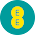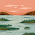### Principle of Working of 3-Phase Synchronous Motor

Synchronous motor works on the principle of the magnetic locking. When two unlike poles are brought near each other, if the magnets are strong, there exists a tremendous force of attraction between those two poles. In such condition the two magnets are said to be magnetically locked.
If now one of the two magnets is rotated, the other also rotates in the same direction, with the same speed due to the force of attraction i.e. due to magnetic locking condition. The principle is shown schematically in the Fig.1.Fig.  1  Principle of magnetic locking

So to have the magnetic locking condition, there must exist two unlike poles and magnetic axes of two must be brought very close to each other. Let us see the application of this principle in case of synchronous motor.
Consider a three phase synchronous motor, whose stator is wound for 2 poles. The two magnetic fields are produced in the synchronous motor by exciting both the windings, stator and rotor with three phase a.c. supply and d.c. supply respectively. When three phase winding is excited by a three phase a.c. supply the the flux produced by the three phase winding is always of rotating type, which is already discussed in the previous post. Such a magnetic flux rotates in space at a speed called synchronous speed. This magnetic field is called rotating magnetic field. The rotating magnetic field creates the effect similar to the physical rotation of magnets in space with a synchronous speed. So stator of the synchronous motor produces one magnet which is as good as rotating in space with the synchronous speed. The synchronous speed of a stator rotating magnetic field depends on the supply frequency and the number of poles for which stator winding is wound. if the frequency of the a.c. supply is f Hz and stator is wound for P number of poles, then the speed of the rotating magnetic field is synchronous given by,
N = 120f/P r.p.m.
In this case, as stator is wound for say 2 poles, with 50 Hz supply, the speed of the rotating magnetic field will be 3000 r.p.m. This effect is similar to the physical rotation of two poles with a speed of N r.p.m. For simplicity of understanding let us assume that the stator poles are N1 and S1 which are rotating at a speed of Ns. The direction of rotation of rotating magnetic field is say clockwise.
When the field winding on rotor is excited by a d.c. supply, it also produces two poles, assuming rotor construction to be two pole, salient type. Let these poles be N2 and S2
Now one magnet is rotating at Ns having poles N1 and S1 while at start rotor is stationary i.e. second magnet is stationary having poles N2 and S2. If somehow the unlike poles N1 and S2 or S1 and N2 are brought near each other, the magnetic locking may get established between stator and rotor poles. As stator poles are rotating due to magnetic locking rotor will also rotate in the same direction as that of stator poles i.e. in the direction of rotating magnetic field, with the same speed i.e Ns. Hence synchronous motor rotates at one and only one speed i.e. synchronous speed. But this all depends on existence of magnetic locking between stator and rotor poles. Practically it is not possible for stator poles to pull the rotor poles from their stationary position into magnetic locking condition. hence synchronous motors are not self starting. Let us see the reason behind this in detail.

1.2.3.